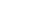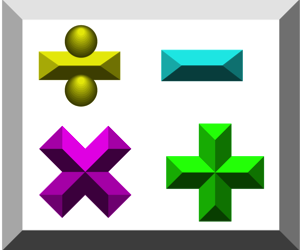• # Welcome!

Open or CloseThis section provides you with FREE video self-tutorials in the area of Basic Mathematics, also known as Pre-Algebra. I start out explaining all about numbers, basic arithmetic, order of operations, fractions and more. Be aware that the tutoring is designed for students from Seventh Grade through College. It is NOT for those in Kindergarten through Sixth Grade.

Watching all these videos is a great way to prepare for Algebra!

*Special Note to Teachers, Parents and Tutors: If you plan to use my videos for teaching/tutoring purposes, you need to buy the DVDs (not just watch the free videos on this page with your students). The good news is no additional school licenses are needed!

Students: Although not required, it is appreciated if you could buy at least one DVD lesson from me. If you cannot do this, then perhaps you can make a small donation to my YouTube Channel.

Your purchases/tips allow me to dedicate more time to creating even MORE and BETTER videos for you! Thank you in advance!

NOTE: Most videos have associated PDF Math Notes or Worksheets. Visit my Printshop for details.
• # Numbers

Open or Close
Basic Math: Lesson 1 - Numbers

This lesson consists of providing you with a Self-Tutorial on all about the classification and sets of numbers. Learn what are natural numbers, integers, rational numbers and more. I also explain how to use your graphing calculator to input all types of numbers (integers, fractions, square roots, etc.).
• # Equalities & Inequalities

Open or Close
Basic Math: Lesson 2 - Equalities & Inequalities

This lesson consists of providing you with a Self-Tutorial on the basics of equalities and inequalities. I go over how to write results in interval notation, inequality notation, and set (set-builder) notation. I also explain in the printed notes how to use your graphing calculator to help you make comparisons between numbers.
• # Operations On Numbers - Part 1

Open or Close
Basic Math: Lesson 3 - Operations On Numbers - Part 1

Part 1 of 3 Videos. This lesson consists of providing you with a Self-Tutorial on the typical operations done on numbers. These include: absolute value, opposites, addition, subtraction, multiplication, division and simple exponents. Order of Operations is covered in detail. I also explain how to use your graphing calculator to help you perform all these operations.
• # Operations On Numbers - Part 2

Open or Close
Basic Math: Lesson 3 - Operations On Numbers - Part 2

Part 2 of 3 Videos. See above link for video description.
• # Operations On Numbers - Part 3

Open or Close
Basic Math: Lesson 3 - Operations On Numbers - Part 3

Part 3 of 3 Videos. See Part 1 link for video description.
• # Properties of Numbers

Open or Close
Basic Math: Lesson 4 - Properties of Numbers

This lesson consists of providing you with a Self-Tutorial of the basic properties of numbers. These are the ones I discuss:

The Reflexive Property of Equality, The Symmetric Property of Equality, The Transitive Property of Equality, The Substitution Property, The Additive Property of Equality, The Cancellation Law of Addition, The Multiplicative Property of Equality, Cancellation Law of Multiplication, The Zero-Factor Property, The Law of Trichotomy, The Transitive Property of Inequality, Properties of Absolute Value, The Closure Property of Addition, The Closure Property of Multiplication, The Commutative Property of Addition, The Commutative Property of Multiplication, The Associative Property of Addition, The Associative Property of Multiplication, The Identity Property of Addition, The Identity Property of Multiplication, The Inverse Property of Addition, The Inverse Property of Multiplication, The Distributive Law of Multiplication Over Addition, The Distributive Law of Multiplication Over Subtraction, The General Distributive Property and The Negation Distributive Property.
• # Factors, Multiples & Divisibility

Open or Close
Basic Math: Lesson 5 - Factors, Multiples & Divisibility

This lesson consists of giving you the necessary "preliminaries" to do fractions. The tutor explains what are factors, divisors, prime and composite numbers, how to do prime factorization, GCFs, LCMs, multiples, and the rules of divisibility. He also explains how to use your graphing calculator to help you find the GCF, LCM and multiples.
• # Sieve of Eratosthenes

Open or Close
Basic Math: Sieve of Eratosthenes

This lesson consists of showing you how to use the Sieve of Eratosthenes to find all prime numbers below a certain value. In this case, below 100.
• # Introduction to Fractions

Open or Close
Basic Math: Lesson 6 - Introduction to Fractions

An informal introduction to why we need to learn about fractions.

The next 12 videos provide you with a Self-Tutorial on fractions. This video series is different from the previous ones in that the use of printed
Notes is integrated with the Video (Math Notes for other lessons may be used independently of the videos). This series is VERY detailed and covers the necessary areas to help students review the many aspects of fractions and fraction conversions. I also show you how to perform all the many different fraction operations and conversions using the TI-84+ Graphing Calculator.
• # Understanding Fractions

Open or Close
Basic Math: Lesson 6 - Video Clip #1 - Understanding Fractions

Concepts covered: Denominator, Numerator, Common Fraction, and Unit Fraction. I also teach you how to draw typical fractions on a circle.
• # Types of Fractions

Open or Close
Basic Math: Lesson 6 - Video Clip #2 - Types of Fractions

Concepts covered: Proper Fraction, Improper Fraction, Mixed Number, and Complex Fraction.
• # Equivalent Fractions

Open or Close
Basic Math: Lesson 6 - Video Clip #3 - Equivalent Fractions

Concepts covered: Like Fractions, Common Denominator, Equivalent Fractions, Reduced Fraction, Lowest Terms (or Simplest Form), The Cancellation Method, The GCF Method, and Higher Form.
• # The LCD & Fraction Comparisons

Open or Close
Basic Math: Lesson 6 - Video Clip #4 - The LCD & Fraction Comparisons

Concepts covered: Least (or Lowest) Common Denominator and learn how to compare the sizes of different fractions.
• # Other Fractional Expressions

Open or Close
Basic Math: Lesson 6 - Video Clip #5 - Other Fractional Expressions

Concepts covered: Decimal Fraction, Percentage, and Ratio.
• # Converting Fractional Expressions

Open or Close
Basic Math: Lesson 6 - Video Clip #6 - Converting Fractional Expressions

Concepts covered: Step-by-Step directions on how to perform (by hand and using the calculator) the following conversions:

Improper Fraction -> Mixed Number
Mixed Number -> Improper Fraction
Common Fraction -> Decimal Form
Terminating Decimal -> Fraction
Repeating Decimal -> Fraction (calculator only-- by hand is covered in Algebra)
Decimal -> Percentage
Common Fraction -> Percentage
Percentage -> Decimal
Percentage -> Common Fraction
Converting Ratios
• # Multiplication of Fractions

Open or Close
Basic Math: Lesson 6 - Video Clip #7 - Arithmetic of Fractions: Multiplication

Concepts covered: Multiplication of Improper and Proper Fractions and also Mixed Numbers.
• # Division of Fractions

Open or Close
Basic Math: Lesson 6 - Video Clip #8 - Arithmetic of Fractions: Division

Concepts covered: Reciprocal, Inverting a Fraction, Division of Improper, Proper and Complex Fractions and Mixed Numbers. Converting Complex Fractions to Simple Fractions is also done here.

Open or Close
Basic Math: Lesson 6 - Video Clip #9 - Arithmetic of Fractions: Addition

Concepts covered: Addition of Improper and Proper Fractions and also Mixed Numbers.
• # Subtraction of Fractions

Open or Close
Basic Math: Lesson 6 - Video Clip #10 - Arithmetic of Fractions: Subtraction

Concepts covered: Subtraction of Improper and Proper Fractions and also Mixed Numbers.
• # Answers to Fraction Quiz

Open or Close
Basic Math: Lesson 6 - Answers to Quiz Questions

Step-by-step answers to the quiz that is at the end of the Lesson Notes.
• # Units of Measurement

Open or Close
Basic Math: Lesson 7 - Units of Measurement

This lesson consists of providing you with a Self-Tutorial of the basic units used in measurement. These are the ones I discuss:
Units of Time, Units of Length (Metric and U.S.), Units of Area (Metric and U.S.), Units of Volume (Metric and U.S.), Units of Mass (Metric and U.S.), Units of Liquid Capacity (Metric and U.S.), Units of Dry Capacity (Metric and U.S.), and other miscellaneous Units. Conversion Formulas and Abbreviations are also listed.

This is a "must have" Lesson for those moving on to solving Word Problems in Algebra and other higher math.
• # Quick Math Review to Prep for Algebra 1

Open or Close
Quick Math Review to Prep for Algebra 1

This is 1 of 4 videos I custom made for an educator in California for an experimental 1-week video homework program. I have only edited the beginning and ending titles. Although not designed for the general public, I figured you may find them useful.

This video provides a quick general math review of concepts needed to do well in Algebra 1.

Covers: Order of Operations, Fraction Arithmetic, Basic Algebraic Concepts and Terminology, Converting Verbal Phrases to Algebraic Expressions and other Miscellaneous Math Concepts.